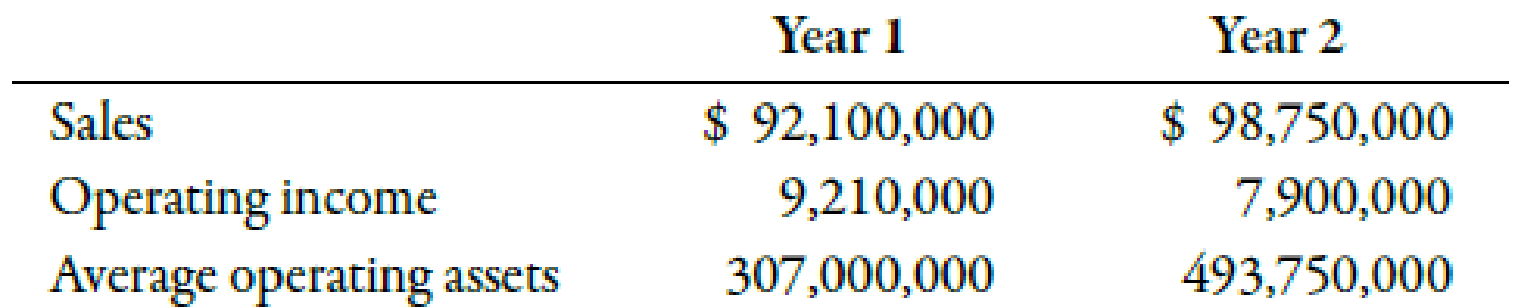Chapter 11, Problem 28E### Managerial Accounting: The Corners...

7th Edition
Maryanne M. Mowen + 2 others
ISBN: 9781337115773

#### Solutions

Chapter
Section### Managerial Accounting: The Corners...

7th Edition
Maryanne M. Mowen + 2 others
ISBN: 9781337115773
Textbook Problem
59 views

# Return on Investment, Margin, TurnoverData follow for the Consumer Products Division of Kisler Inc.:(Note: Round all answers to two decimal places.)Required: 1. Compute the margin and turnover ratios for each year. 2. Compute the ROI for the Consumer Products Division for each year.

1.

To determine

Calculate margin and turnover ratios for each year.

Explanation

Margin:

The Proportion of operating income to the amount of sales revenue is known as margin. It represents the proportion of sales revenue left, to cover taxes, interest, and profit.

Turnover:

Turnover can be defined as the amount of dollar sales earned by the company from investing every dollar in the operating assets. It is computed by dividing the amount of sales by the average operating assets.

The following table represents the calculation of the margin:

 Year 1 ($) Year 2 ($) Operating income (A) 9,210,000 7,900,000 Sales (B) 92,100,000 98,750,000 Margin (AB) 0

2.

To determine

Calculate ROI for each year.

### Still sussing out bartleby?

Check out a sample textbook solution.

See a sample solution

#### The Solution to Your Study Problems

Bartleby provides explanations to thousands of textbook problems written by our experts, many with advanced degrees!

Get Started

#### Find more solutions based on key concepts